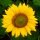# RECIPE. LaTeX + gnuplot (tight box EPS) + maxima

Here is how you can get gnuplot (and a little maxima) to work within LaTeX documents:

License: GPL 3 or later (remember to cite).

It requires gnuplot 4.4 (from GNU ppa), maxima 5.24 (from Trisquel 6.0 repo), ps2eps 1.68 (from Trisquel 6.0 repo), pdfTeX 3.1415926-1.40.10-2.2 (from Trisquel 6.0 repo), dvips(k) 5.98 (from Trisquel 6.0 repo), and the corresponding LaTeX packages (amsmath, graphicx, color, hyperref*, caption* and cleveref*)[*: optional]

First, create a document with the following content:

\documentclass[a4paper]{article}

\usepackage{amsmath}
\usepackage{graphicx} % for eps files
\usepackage[hypcap]{caption} % link point to the top of the image
\usepackage{color}
% text corresponding to the target's type

%% will prepend the section number to all equation numbers (amsmath)
\numberwithin{equation}{section}

%% Documentation to execute bash:
%%tex.stackexchange.com/questions/14186/how-can-i-provide-a-verbatim-unescaped-commandline-for-executing-with-write18
\def\exec{\begingroup\setexeccatcodes\innerexec}
\def\setexeccatcodes{\catcode\#=12 \catcode\%=12{}
\catcode\=12 \catcode\$=12 \catcode\^=12 \catcode\\=12 } \def\innerexec#1{\immediate\write18{\unexpanded{#1}}\endgroup} \def\leer{\begingroup\setleercatcodes\innerleer} \def\setleercatcodes{\endlinechar=-1} \def\innerleer#1{\immediate\input{|#1}\endgroup} % Function to get raw code and generate EPS \newcommand\gnuplotcmd{% arg 1: commands; arg 2: name of output file \exec{echo 'set terminal epslatex color colortext standalone; set output "#1.tex"; #2; set output;' | gnuplot;} \exec{latex "#1.tex" && dvips "#1.dvi" && ps2eps -f -q "#1.ps" && mv "#1.eps" "../Figures/#1.eps"; rm -f "#1".tex "#1".dvi ../Figures/"#1".tex; } } % Command to run gnuplot code and insert the result as picture (tight) \newcommand\gnuplot{ % First argument: caption. Second argument: name of file (and figure). Third % argument: code % (all the commands are sent as a single line) \gnuplotcmd{#2}{#3} %% Make picture tight with epstool: %\exec{epstool --bbox --quiet --copy "#3".eps "#3".eps.mod} %\exec{mv "#3".eps.mod "../Figures/""#3".eps} \centering \includegraphics{../Figures/#2.eps} \caption{#1\label{fig:#2}} } % Command to run gnuplot code and insert the result as picture (tight) \newcommand{\gnuplotfile}{ % arg 1: caption; arg 2: name of eps file (and label); % arg 3: file with gnuplot code % Add a line to replot and send to a file in gnuplot \exec{echo 'load "#3"; set terminal epslatex color colortext standalone; set output "#2.tex"; replot; set output;' | gnuplot} \exec{latex "#2.tex" && dvips "#2.dvi" && ps2eps -f -q "#2.ps" && mv "#2.eps" "../Figures/#2.eps"; rm -f #2.tex #2.dvi; } % Add picture with caption and label \centering \includegraphics{../Figures/#2.eps} \caption{#1\label{fig:#3}} } % Command to execute maxima code and print it as LaTeX \newcommand\maxima{ %%$ \displaystyle\maxima{diff(f(x),x)} $%% Documentation %% tex.stackexchange.com/ questions/ 87095/ is-it-possible-to-write-all-mathematical-formulas-in-a-separate-file- and-add-the \exec{ maxima --very-quiet % remove headings from maxima -r ' % read commands load("mactex-utilities")'\$' % translations from tex to latex
display2d: false'\$' % no fancy notation tex(#1)'\$ |  % convert to tex in maxima
tr -d \$| % remove dollar signs sed '/^'\$'/{d}' > /tmp/maxima.aux % dump output to file
}
}

\begin{document}
% Create a dedicated folder for the Figures
\exec{[ ! -d ../Figures ] && mkdir ../Figures}

Write here

\end{document}


Then run the following:

 latex --synctex=1 --shell-escape --file-line-error-style YOURFILE && dvips YOURFILE && ps2pdf YOURFILE.ps

(you should only execute latex --shell-escape with files that you trust; malware can live in TEX files.) It is possible that the references are not there the first time. You may need to run the latex command two times. Also don't forget to save the document something like LatexDocument.tex (YOURFILE=LatexDocument, without extension). Also, don't forget to finish every line of the gnuplot code with semicolon.

The following is an example (replace "Write here" in the above code

% Normal LaTeX equation (it will look nice, but not always make sense)
\begin{equation} \label{eq:GeneralVolumeIntegral}
V=\int {\int {f\left(x , y , z\right)}{\;z}}{\;dA\left(x , y
\right)}
\end{equation}

% example to use maxima notation:
\begin{subequations} \label{ChgEllipCoord}
\begin{align}
\maxima{r^2 = (x/a)^2 + (y/b)^2} \\
\maxima{x = a*r*cos(\theta)} \label{ChgEllipCoord1}\\
\maxima{y = b*r*sin(\theta)} \label{ChgEllipCoord2}
\end{align}
\end{subequations}

This is a derivative with maxima code: $\displaystyle \maxima{diff(f(x),x))}$

% Example gnuplot code (finish every line with semicolon):
\begin{figure}[htb!]
\gnuplot{Caption}{test}{
set xlabel "$x$";
splot tan(x), sin(x);
}
\end{figure}
The figure depicted in \Cref{fig:test} is made with Gnuplot

%% Uncomment and change path to code to test loading from file:
%\begin{figure}[htb!]
%	\gnuplotfile{Caption
%	}{EllipticalFrustum}{path/file.gp}
%\end{figure}

\begin{figure}[htb!]
\centering
\gnuplotcmd{FileGnuplot}{
% Example from the documentation demo_4.4/contours.1.gnu
set samples 20, 20;
set isosamples 21, 21;
set contour base;
set title "3D gnuplot demo - contour plot";
set xlabel "X axis";
set ylabel "Y axis";
set zlabel "Z axis";
set zlabel  offset character 1, 0, 0 font "" textcolor lt -1 norotate;
splot x*y;
}
\includegraphics[]{../Figures/FileGnuplot.eps}
\caption{My Caption \label{label}}
\end{figure}


The attached files contain this example (within the tar.gz file) and the result (tmp.pdf)

There are other alternatives, also:

An attachment has a modified version from J.M. Mira's work to improve working with maxima in subequations, adds a faster version for single equations and fixes some gnuplot functionalities. To open the tar files, use xarchiver, file-roller or run tar -xzf in the terminal.

Notice that if you write \theta or theta, you may get \vartheta (as defined by maxima) in your final document, and \\theta will get you \theta (as defined by maxima). Play around with pi, \pi and \\pi as well. If you prefer \theta over \vartheta, and would like cos, sin, tan and other functions to have parentheses like cos(), you can check the files in fullexample.tar_.gz (with xarchiver, file-roller or tar -xzf) for a patch and modified files. View the README file.

For the very VERY brave there is a full example for Maxima 5.29 to include gnuplot running from maxima, and loading LaTeX symbols.

AttachmentSize
tmp.pdf42.8 KB
tmp.tar_.gz2.07 KB
maxiplot-tmp.tar_.gz54.87 KB
fullexample.tar_.gz37.17 KB
fullexample5.29.tar_.gz163.91 KB

### Revisions

09/04/2013 - 20:15